| Table of Contents | Function Reference | Function Finder | R Project |

SIMPLE NONLINEAR CORRELATION AND REGRESSION

Preliminaries

Some new tools will be introduced in this tutorial for dealing with nonlinear relationships between a single numeric response variable and a single numeric explanatory variable. In most cases, this involves finding some way to transform one or both of the variables to make the relationship linear. Thereafter, the methods follow those of Simple Linear Correlation and Regression. You might want to review that tutorial first if you are not comfortable with those methods.

Although it isn't strictly necessary at this time, as regression problems become more complex, it will become increasingly necessary for you to read the Model Formulae tutorial, as the model to be fit and tested by R will have to be specified by a model formula. You can probably skip it for now, but you'll have to read it if you go any further than this.

Kepler's Law

It took Johannes Kepler about ten years to find his third law of planetary motion. Let's see if we can do it a little more quickly. We are looking for a relationship between mean solar distance and orbital period. Here are the data. (We have a little more than Kepler did back at the beginning of the 17th century!)

```planets = read.table(header=T, row.name=1, text="
planet    dist   period
Mercury   57.9    87.98
Venus    108.2   224.70
Earth    149.6   365.26
Mars     228.0   686.98
Ceres    413.8  1680.50
Jupiter  778.3  4332.00
Saturn  1427.0 10761.00
Uranus  2869.0 30685.00
Neptune 4498.0 60191.00
Pluto   5900.0 90742.00
")```
The row.names=1 option should put the column labeled "planet" (the 1st column) into the row names of our data frame. Thus, we have two numeric variables: dist(ance) from the sun in millions of kilometers, orbital period in earth days. I'm going to begin with a suggestion, which is to convert everything to units of earth orbits. Thus, the units of distance will become AUs, and the units of period will become earth years.
```> planets\$dist = planets\$dist/149.6
> planets\$period = planets\$period/365.26
> planets
dist      period
Mercury  0.3870321   0.2408695
Venus    0.7232620   0.6151782
Earth    1.0000000   1.0000000
Mars     1.5240642   1.8807972
Ceres    2.7660428   4.6008323
Jupiter  5.2025401  11.8600449
Saturn   9.5387701  29.4612057
Uranus  19.1778075  84.0086514
Neptune 30.0668449 164.7894650
Pluto   39.4385027 248.4312544```
Now a nice scatterplot is in order.
`> with(planets, scatter.smooth(period ~ dist, span=7/8, pch=16, cex=.6, las=1))`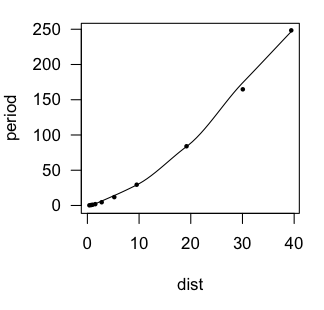In the scatter.smooth() function, "span=" sets a loess smoothing parameter, "pch=" sets the point character for the plot (16 = filled circles), "cex=" sets the size of the points (6/10ths of default), and "las=1" turns the numbers on the axis to a horizontal orientation. We can see that the loess line is a bit lumpy, as loess lines often are, but it is definitely not linear.

An accelerating curve such as this one suggests either an exponential relationship or a power relationship. To get the first, we would log transform the response variable. To get the second, we would log transform both variables. Let's try that and see if either one gives us a linear relationship.

```> with(planets, scatter.smooth(log(period) ~ dist))
> title(main="exponential")
> with(planets, scatter.smooth(log(period) ~ log(dist)))
> title(main="power")```
I'm gonna go out on a limb here and guess that the exponential relationship is not the one we want. The shape of that curve suggests that we need an additional log transform of the explanatory variable, which is what we have with the power function. The power relationship looks pretty much linear. Let's go with that.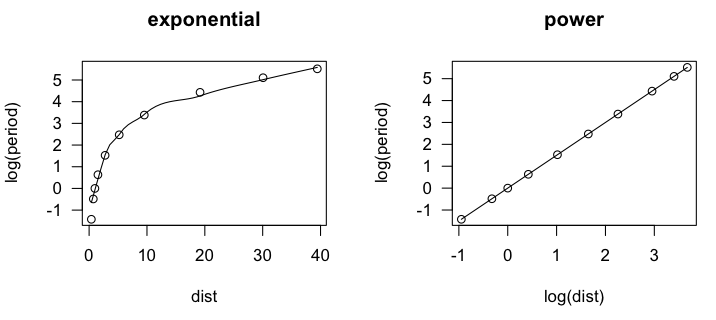```> lm.out = lm(log(period) ~ log(dist), data=planets)
> summary(lm.out)

Call:
lm(formula = log(period) ~ log(dist), data = planets)

Residuals:
Min         1Q     Median         3Q        Max
-0.0011870 -0.0004224 -0.0001930  0.0002222  0.0022684

Coefficients:
Estimate Std. Error  t value Pr(>|t|)
(Intercept) -0.0000667  0.0004349   -0.153    0.882
log(dist)    1.5002315  0.0002077 7222.818   <2e-16 ***
---
Signif. codes:  0 '***' 0.001 '**' 0.01 '*' 0.05 '.' 0.1 ' ' 1

Residual standard error: 0.001016 on 8 degrees of freedom
Multiple R-squared:      1,	Adjusted R-squared:      1
F-statistic: 5.217e+07 on 1 and 8 DF,  p-value: < 2.2e-16```
The residuals are virtually zero, as is the intercept, and R-squared is 1. I'm guessing we've nailed this, but let's take a look at a residual plot to see if we have left any curvilinear trend unaccounted for.
`> plot(lm.out, 1)`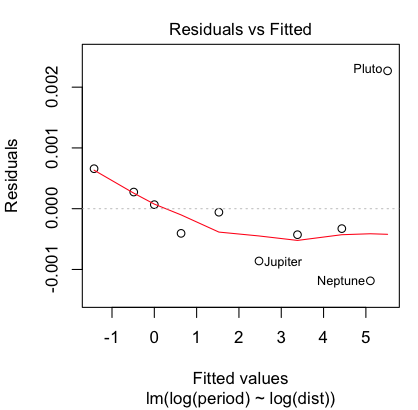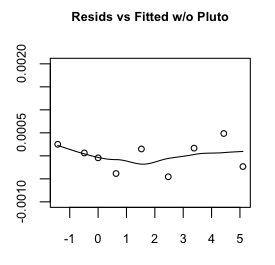Hmmm, maybe a little bit, but that's mostly due to the oddball (outlier) Pluto, which does have a somewhat eccentric and inclined orbit. Even so, the size of the residuals is miniscule, so I'm going to say our regression equation is:log(period) = 1.5 log(dist)

Exponentiating and squaring both sides brings us to the conclusion that the square of the orbital period (in earth years) is equal to the cube of the mean solar distance (actually, semi-major axis of the orbit, in AUs). Had we left the measurements in their original units, we'd also have constants of proportionality to deal with, but all things considered, I think we've done a sterling job of replicating Kepler's third law, and in under an hour as well. If only Johannes had had access to R!

Sparrows in the Park

Notice in the last problem the relationship between the response and explanatory variables was not linear, but it was monotonic. Such accelerating and decelerating curves suggest logarithmic transforms of one or the other or both variables. Relationships that are nonmonotonic have to be modeled differently.

The following data are from Fernandez-Juricic, et al. (2003). The data were estimated from a scatterplot presented in the article so is not exactly the same data the researchers analyzed but is close enough to show the same effects.

```sparrows = read.table(header=T, text="
pedestrians pairs
1.75  14.2
5.83  30.1
5.33  71.2
4.67  77.5
7.17  75.9
5.50 121.8
9.33 132.1
6.83 159.0
7.50 181.9
10.80 184.3
11.30 194.6
11.40 219.1
10.50 246.8
12.80 166.9
13.80 162.2
17.80 134.5
16.20  94.1
19.80  88.6
21.90  90.2
26.60  44.3
28.70  14.2
32.60  15.8
38.20  47.5
")```
The study examined the effect of human disturbance on the nesting of house sparrows (Passer domesticus). Breeding pairs of birds ("pairs") per hectare were counted in 23 parks in Madrid, Spain. They also counted the number of people walking through the park per hectare per minute ("pedestrians"). The relationship is nonlinear and nonmonotonic, as shown by a scatterplot. (The black line is the lowess line. The red line will be explained below.)
`> with(sparrows, scatter.smooth(pairs ~ pedestrians))`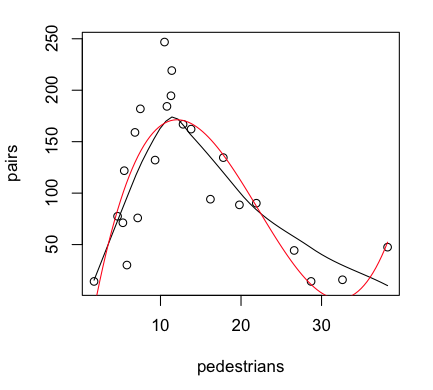We will model this relationship with a polynomial regression equation, and I would say from looking at the scatterplot that the equation ought to, at least initially, include a cubic term.
```> lm.out = lm(pairs ~ pedestrians + I(pedestrians^2) + I(pedestrians^3), data=sparrows)
> summary(lm.out)

Call:
lm(formula = pairs ~ pedestrians + I(pedestrians^2) + I(pedestrians^3),
data = sparrows)

Residuals:
Min      1Q  Median      3Q     Max
-80.756 -17.612   0.822  20.962  78.601

Coefficients:
Estimate Std. Error t value Pr(>|t|)
(Intercept)      -91.20636   40.80869  -2.235   0.0376 *
pedestrians       49.69319    8.63681   5.754 1.52e-05 ***
I(pedestrians^2)  -2.82710    0.50772  -5.568 2.27e-05 ***
I(pedestrians^3)   0.04260    0.00856   4.977 8.37e-05 ***
---
Signif. codes:  0 '***' 0.001 '**' 0.01 '*' 0.05 '.' 0.1 ' ' 1

Residual standard error: 39.31 on 19 degrees of freedom
Multiple R-squared:  0.7195,	Adjusted R-squared:  0.6752
F-statistic: 16.24 on 3 and 19 DF,  p-value: 1.777e-05```
The cubic term is significant, so if we are going to retain it, we should retain the lower order terms as well. (NOTE: In the formula interface, we cannot simply enter pedestrians^2 and pedestrians^3. Inside a formula interface, the ^ symbol means something else. If we want it to mean exponentiation, we must isolate it from the rest of the formula using I().) Our regression equation appears to be:

pairs-hat = -91.2 + 49.7 * pedestrians - 2.83 * pedestrians^2 + 0.0426 * pedestrians^3

Provided the graphics device is still open with the scatterplot showing, we can plot the regression curve on it as follows (the red line in the graph above).

`> curve(-91.2 + 49.7*x - 2.83*x^2 + 0.0426*x^3, add=T, col="red")`
Notice that the regression line picks up that last point better than the lowess line does. And therein lies a problem.
`> plot(lm.out, 4)                 # Cook's Distance plot`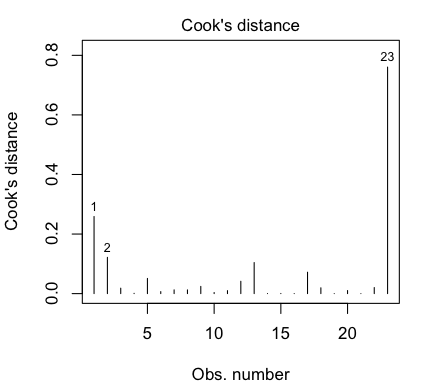That last point is a highly influential point, with a Cook's Distance of nearly 0.8. We really don't like to have individual points having a strong influence over the shape of our regression relationship. Perhaps we should check the relationship with that point deleted, which we can do most conveniently using the update() function to update the data set that we used. (We can also use update() to add or remove variables, add or remove effects such as interactions, add or remove an intercept term, and so on. More on this in the next tutorial.)

```> lm.out2 = update(lm.out, data=sparrows[-23,])       # delete case 23
> summary(lm.out2)                                    # output not shown```
I'm not going to go too far down this road, because it's a bumpy road. As a final comment I'll say that sometimes you have to go with what the phenomenon suggests rather than what the statistics suggest, and in this case the authors concluded that a quadratic model was most appropriate. They reasoned that, at first, as human activity increases, nesting activity also increases, because humans mean food. But when human activity gets too high, nesting activity is disrupted.

• Fernandez-Juricic, E., Sallent, A., Sanz, R., and Rodriguez-Prieto, I. (2003). Testing the risk-disturbance hypothesis in a fragmented landscape: non-linear responses of house sparrows to humans. Condor, 105, 316-326.

Vapor Pressure of Mercury

In this section we are going to use a data set called "pressure".

```> data(pressure)
> str(pressure)
'data.frame':   19 obs. of  2 variables:
\$ temperature: num  0 20 40 60 80 100 120 140 160 180 ...
\$ pressure   : num  2e-04 0.0012 0.006 0.03 0.09 0.27 0.75 1.85 4.2 8.8 ...
> summary(pressure)
temperature     pressure
Min.   :  0   Min.   :  0.0002
1st Qu.: 90   1st Qu.:  0.1800
Median :180   Median :  8.8000
Mean   :180   Mean   :124.3367
3rd Qu.:270   3rd Qu.:126.5000
Max.   :360   Max.   :806.0000```
The two variables are "temperature" in degrees Celsius, and the vapor "pressure" of mercury in millimeters of mercury. This built-in data set is derived from the Handbook of Chemistry and Physics, CRC Press (1973). A brief explanation may be in order for my less physical-science-oriented colleagues. When a liquid is placed in an evacuated, closed chamber (i.e., in a "vacuum"), it begins to evaporate. This vaporization will continue until the vapor and the liquid reach a dynamic equilibrium (the number of particles of liquid that go into the vapor is equal to the number of particles of vapor that go into the liquid). At this point, the pressure exerted by the vapor on the liquid is called the vapor pressure (or equilibrium vapor pressure) of the substance. Vapor pressure has a complex relationship with temperature, and so far as I know, there is no law in theory that specifies this relationship (at least not in the case of mercury). Since we are dealing with a liquid/vapor phase transition, and not just a gas, the simple gas laws you learned back in high school chemistry don't apply. The vapor pressure of mercury and its relationship to temperature has been an important question, for example, in the calibration of scientific instruments such as mercury thermometers and barometers.

To bring this problem into the 21st century, I am going to begin by converting the units of measurement to SI units. This will also avoid some problems with log transforms. (Notice the minimum value of "temperature" is 0. Notice also that the number of significant figures present in the various data values varies widely, so we are starting off with a foot in the bucket.)

```> pressure\$temperature = pressure\$temperature + 273.15
> ### temperature is now in degrees kelvin
> pressure\$pressure = pressure\$pressure * .1333       # .133322365 would be more accurate
> ### pressure is now in kiloPascals
> summary(pressure)
temperature       pressure
Min.   :273.1   Min.   :2.666e-05
1st Qu.:363.1   1st Qu.:2.399e-02
Median :453.1   Median :1.173e+00
Mean   :453.1   Mean   :1.657e+01
3rd Qu.:543.1   3rd Qu.:1.686e+01
Max.   :633.1   Max.   :1.074e+02```
These are linear transformations and will not affect the nature of any relationship we might find. Now, it annoys me to have to type something like pressure\$pressure, so I would like to attach this data frame. But that produces an annoying warning of a conflict, because one of the variables in the data frame has the same name as the data frame. So, we will extract the variables from the data frame, putting them in the workspace with more "typeable" names. This will make it unnecessary to reference the data frame, and yet if we get careless and mess something up, we still have the data frame as a backup.
```> pres = pressure\$pressure
> temp = pressure\$temperature
> ls()
 "pres"     "pressure" "temp"```
And we are finally ready to go.

First Thoughts: Log Transformations

We begin, as always, with a scatterplot.

```> par(mfrow=c(1,4))                    # one row of four graphs
> plot(pres ~ temp, main="Vapor Pressure\nof Mercury",
+                   xlab="Temperature (degrees Kelvin)",
+                   ylab="Pressure (kPascals)")```
When I see something like that (leftmost graph), I immediately think "exponential relationship," so a log(y) transform might be in order. This can be done in two ways: by actually transforming the variable to create a new variable by logpres=log(pres), or by having R plot the relationship with a logarithmic axis.
```> plot(pres ~ temp, main="Vapor Pressure\nof Mercury",
+                   xlab="Temperature (degrees Kelvin)",
+                   ylab="Pressure (kPascals)", log="y")```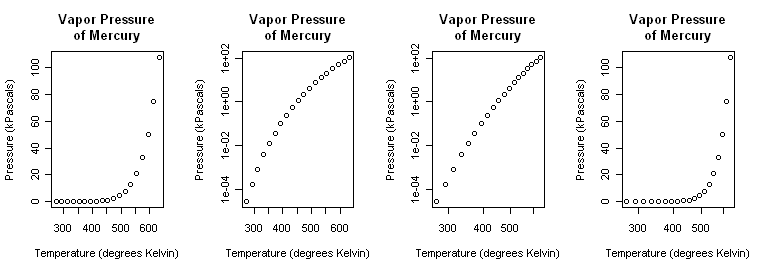No (second graph), that appears to have overcorrected. So let's try a power function (log(y) and log(x) transforms).
```> plot(pres ~ temp, main="Vapor Pressure\nof Mercury",
+                   xlab="Temperature (degrees Kelvin)",
+                   ylab="Pressure (kPascals)", log="xy")```
That (third graph) appears to be a little closer but still a bit bowed. A log(x) transform (last graph) is silly but illustrated just to show it.
```> plot(pres ~ temp, main="Vapor Pressure\nof Mercury",
+                   xlab="Temperature (degrees Kelvin)",
+                   ylab="Pressure (kPascals)", log="x")```
Close the graphics window now so that it will reset to the default one-graph per window partition. (A trick: After par() opened a graphics window, I resized it by hand to a long, skinny window.)

Although we know it's wrong from the scatterplots, let's fit linear models to the exponential and power transforms just to illustrate the process.

```> ls()                                 # just checking
 "pres"     "pressure" "temp"
> par(mfrow=c(1,2))                    # one row of two graphs
> lm.out1 = lm(log(pres) ~ temp)       # the exponential model
> lm.out1

Call:
lm(formula = log(pres) ~ temp)

Coefficients:
(Intercept)         temp
-18.95245      0.03979

> plot(lm.out1\$fitted, lm.out1\$resid)  # evaluated via a residual plot; plot(lm.out1,1) also works
>
> lm.out2 = lm(log(pres) ~ log(temp))  # the power model
> lm.out2

Call:
lm(formula = log(pres) ~ log(temp))

Coefficients:
(Intercept)    log(temp)
-108.11        17.61

> plot(lm.out2\$fitted, lm.out2\$resid)  # evaluated via a residual plot; or plot(lm.out2,1)```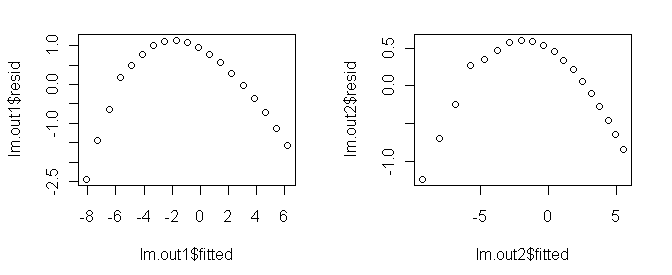The residual plots show a clear nonrandom pattern, betraying the fact that we have the wrong models here. We have missed a substantial amount of the curvilinear relationship.

Polynomial Regression

A polynomial of sufficient order will fit any scatterplot perfectly. If the order of the polynomial is one less than the number of points to be fitted, the fit will be perfect. However, it will certainly not be useful or have any explanatory power. Let's see what we can do with lower order polynomials.

A glance at the scatterplot is enough to convince me that this is not a parabola, so let's start with a third order polynomial.

```> ls()                                 # always good to know what's there
 "lm.out1"  "lm.out2"  "pres"     "pressure" "temp"
> options(show.signif.stars=F)         # turn off annoying significance stars
> lm.out3 = lm(pres ~ temp + I(temp^2) + I(temp^3))
> summary(lm.out3)

Call:
lm(formula = pres ~ temp + I(temp^2) + I(temp^3))

Residuals:
Min      1Q  Median      3Q     Max
-4.3792 -2.7240 -0.3315  2.5918  6.1815

Coefficients:
Estimate Std. Error t value Pr(>|t|)
(Intercept) -4.758e+02  6.580e+01  -7.232 2.92e-06
temp         3.804e+00  4.628e-01   8.219 6.16e-07
I(temp^2)   -9.912e-03  1.050e-03  -9.442 1.06e-07
I(temp^3)    8.440e-06  7.703e-07  10.957 1.48e-08

Residual standard error: 3.414 on 15 degrees of freedom
Multiple R-squared: 0.9892,     Adjusted R-squared: 0.987
F-statistic: 456.4 on 3 and 15 DF,  p-value: 5.89e-15

> par(mfrow=c(1,1))                    # unnecessary if this has already been reset
> plot(lm.out3\$fitted, lm.out3\$resid)  ### output not shown```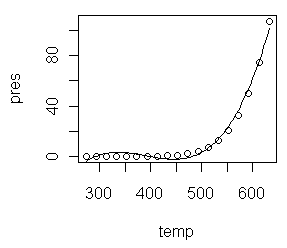Let me explain the model formula first. Three terms are entered into the model as predictors: temp, temp squared, and temp cubed. The squared and cubed terms MUST be entered using "I", which in a model formula means "as is." This is because the caret symbol inside a formula does not have its usual mathematical meaning. If you want it to be interpreted mathematically, which we do here, it must be protected by "I", as in the term "I(temp^2)". This gives the same result as if we had created a new variable, tempsquared=temp^2, and entered tempsquared into the regression formula. (WARNING: If you have read the tutoral on model formulae, you might be tempted to think that pres~poly(temp,3) would have produced the same result. IT DOES NOT!)

All three estimated coefficients are making a signficant contribution to the fit (and the intercept is significantly different from zero as well), and any social scientist would be impressed by an R2-adjusted of 0.987, but the residual plot shows we do not have the right model. To see why, do this.

```> plot(pres~temp)
> curve(-475.8 + 3.804*x - .009912*x^2 + .00000844*x^3, add=T)```
This plot is shown above right, and as you can see, the cubic regression equation has distinct inflection points. Our mercury vapor data do not. Increasing the order of the polynomial is a straighforward extension of the above, and it will improve the fit, but it will not result in the correct model. (A fourth order polynomial would be a reasonable try.)

Box-Cox Tranformations

When you get desperate enough to find an answer, you'll eventually start reading the literature! An article I found from Industrial & Engineering Chemistry Reseach (Huber et al., 2006, Ind. Eng. Chem. Res., 45 (21), 7351-7361) suggested to me that a reciprocal transform of the temperature might be worth a try.

`> plot(pres ~ I(1/temp))              # output not shown`
Clearly, that alone is not sufficient to get us to a linear relationship. (Note: Once again, the reciprocal of "temp" had to be protected inside the formula, because in a formula, 1 is not the numeral one. It stands for the intercept, which would make no sense in this context. We could also have done temprecip=1/temp and used temprecip in the formula, i.e., plot(pres~temprecip).)

Box-Cox transformations allow us to find an optimum transformation of the response variable using maximum-likelihood methods.

```> library("MASS")
> par(mfrow=c(1,2))
> boxcox(pres ~ I(1/temp))                            # basic box-cox plot
> boxcox(pres ~ I(1/temp), lambda=seq(-.2,.2,.01))    # finer resolution on lambda
> par(mfrow=c(1,1))```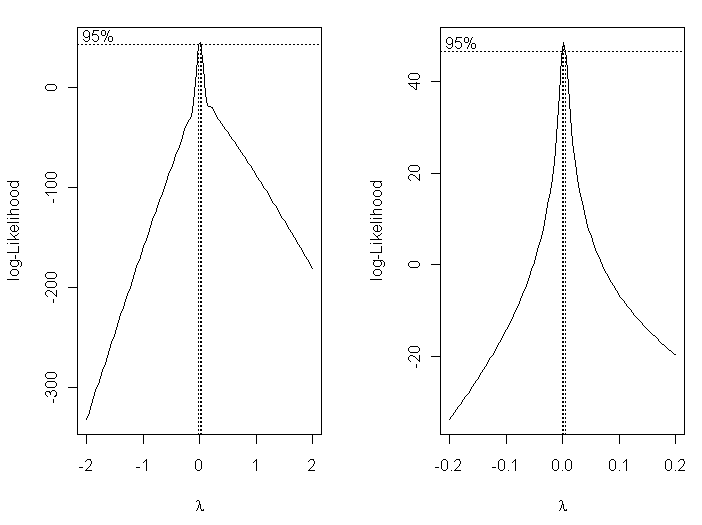The plots suggest we adopt a lambda value very close to zero, which is to say, we should use a log transform of the response variable.
`> plot(log(pres) ~ I(1/temp))`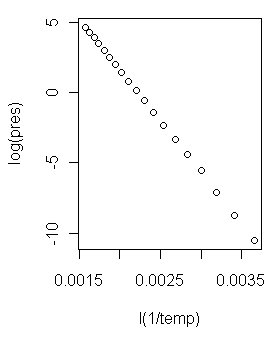Now that is a scatterplot I can live with! (At right.) On with the modeling!
```> lm.out4 = lm(log(pres) ~ I(1/temp))
> summary(lm.out4)

Call:
lm(formula = log(pres) ~ I(1/temp))

Residuals:
Min        1Q    Median        3Q       Max
-0.074562 -0.029292 -0.002077  0.021496  0.151712

Coefficients:
Estimate Std. Error t value Pr(>|t|)
(Intercept)  1.626e+01  4.453e-02   365.1   <2e-16
I(1/temp)   -7.307e+03  1.833e+01  -398.7   <2e-16

Residual standard error: 0.04898 on 17 degrees of freedom
Multiple R-squared: 0.9999,     Adjusted R-squared: 0.9999
F-statistic: 1.59e+05 on 1 and 17 DF,  p-value: < 2.2e-16```
I'd say we might be on to something here! It appears we have a model regression equation as follows.

log(pres) = 16.26 - 7307 / temp

And a look at the regression diagnostic plots...
```> par(mfrow=c(2,2))
> plot(lm.out4)
> par(mfrow=c(1,1))```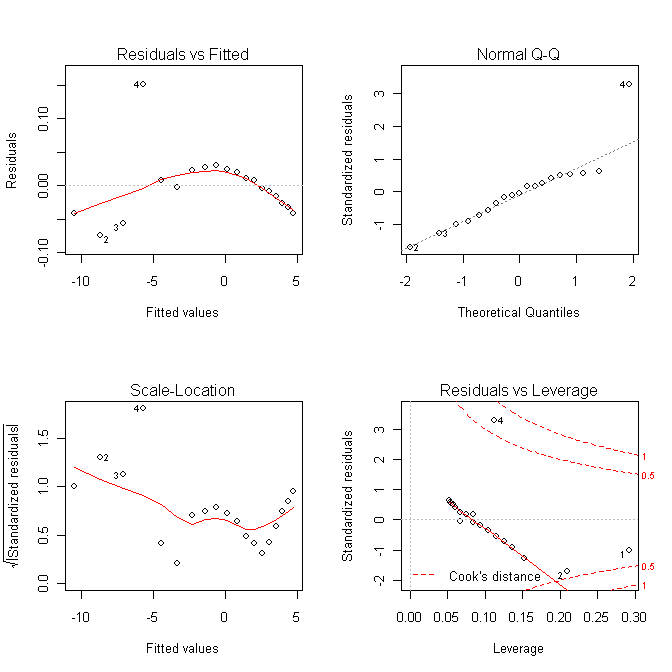...suggests we are still not dead-bang right on, but we are awfully gosh darn close! It might be profitable to toss out case 4 and try again.

Making Predictions From a Model

Predictions for new values of the explanatory variables are made using the predict() function. The syntax of this function makes absolutely no sense to me whatsoever, but I can tell you how to use it to make predictions from a model. We'll use the previous example as our model that we want to predict from. First some reference values...

Values from Handbook of Chemistry and Physics, 76e
Temp (degrees K)400450500550600
Pres (kPa)0.1381.0455.23919.43857.64

...and now we will make predictions from our model for those tabled values of temperature.

```> new.temps = seq(from=400, to=600, by=50)       # put the new values in a vector
> predict(lm.out4, list(temp=new.temps))
1           2           3           4           5
-2.00803385  0.02159221  1.64529305  2.97377556  4.08084431```
The first thing you feed the predict() function is the name of the model object in which you've stored your model. Then give it a list of vectors of new values for the explanatory variable(s) in the format you see above. The output is not pressure in kPa, because that is not what was on the left side of our model formula. The output is log(pres) values. To get them back to actual pressures, we have take the natural antilog of them.
```> exp(predict(lm.out4, list(temp=new.temps)))
1          2          3          4          5
0.1342524  1.0218270  5.1825284 19.5656516 59.1954283```
Those values are not so terribly far from the ones in the table. Nevertheless, I have probably just been expelled from the fraternity of chemical engineers! (Not too bad though, considering that some of our measurements had only one significant figure to begin with.)

revised 2016 February 16
| Table of Contents | Function Reference | Function Finder | R Project |